# [introduction to Python visualization]: 12 small examples of complete data visualization, taking you to play with visualization ~

2022-01-29 17:26:26

in total 22 Complete pyecharts Example , Contains common configuration methods , Each small example contains complete code , To avoid confusion , Each example contains almost a single configuration of code .## 1. Histogram stack

Different series of data use the same stack The values are stacked together ;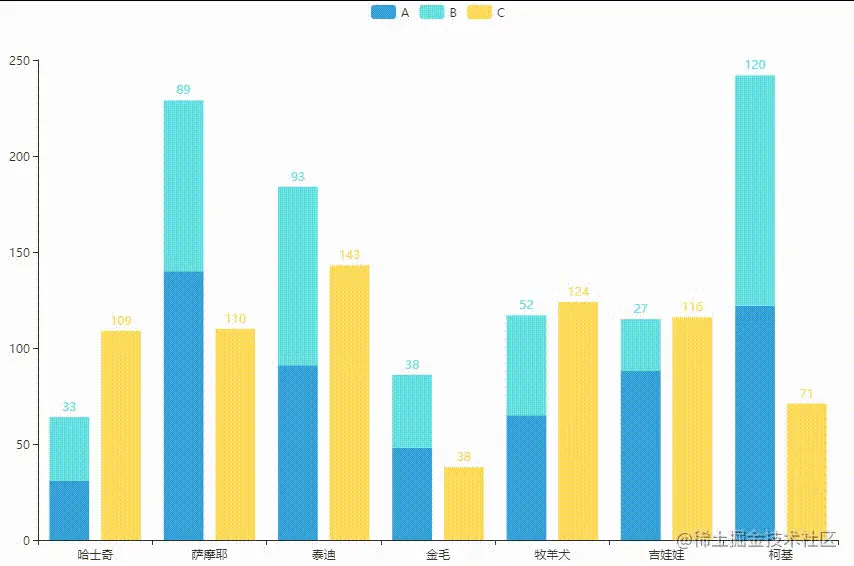``````from pyecharts.charts import *
from pyecharts import options as opts
from pyecharts.faker import Faker

def bar_stack():
bar = Bar(init_opts=opts.InitOpts(theme='light',
width='1000px',
height='600px'))
# stack Series with the same value will be stacked together
return bar

chart = bar_stack()
chart.render_notebook()
Copy code ``````

## 2. Turn off axis display

When the class target signature is too long , You can choose to turn off the axis , Put the data & Labels are displayed directly in the drawing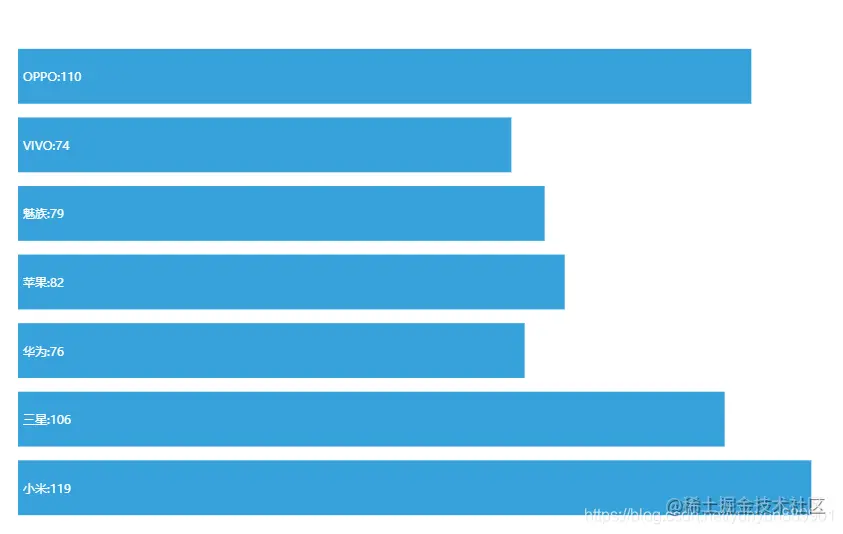``````from pyecharts.charts import *
from pyecharts import options as opts
from pyecharts.faker import Faker

def bar_with_axis_off():
bar = Bar(init_opts=opts.InitOpts(theme='light',
width='1000px',
height='600px'))
#  When the class target signature is too long , You can choose to turn off the axis , Display directly in the drawing
bar.set_series_opts(label_opts=opts.LabelOpts(position='insideLeft', formatter='{b}:{c}'))
bar.set_global_opts(xaxis_opts=opts.AxisOpts(is_show=False),
yaxis_opts=opts.AxisOpts(is_show=False))
bar.reversal_axis()
return bar

chart = bar_with_axis_off()
chart.render_notebook()
Copy code ``````

## 3. Change the axis data type

x The default axis data type is discrete , When using scatter chart, it can be adjusted to numerical type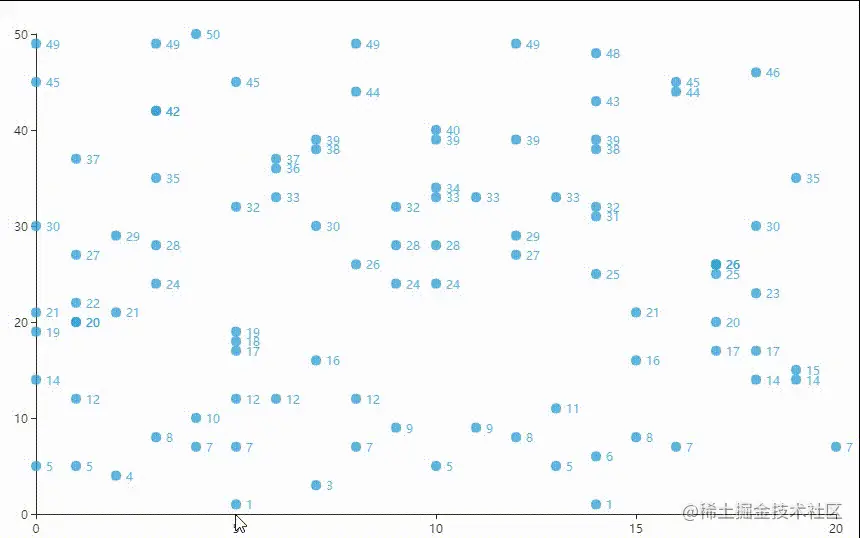``````from pyecharts.charts import *
from pyecharts import options as opts
import random

x_data = [random.randint(0, 20) for _ in range(100)]
y_data = [random.randint(0, 50) for _ in range(100)]

def scatter_with_value_xaxis():
scatter = Scatter(init_opts=opts.InitOpts(theme='light',
width='1000px',
height='600px'))
# X The default axis data type is discrete data , Set to numeric
scatter.set_global_opts(xaxis_opts=opts.AxisOpts(type_="value"))
return scatter

chart = scatter_with_value_xaxis()
chart.render_notebook()
Copy code ``````

## 4. double Y Axis 【 Histogram & Broken line diagram 】

the truth is that Bar and Line Two charts share the same coordinate system , take Bar and Line Point to different Y Axis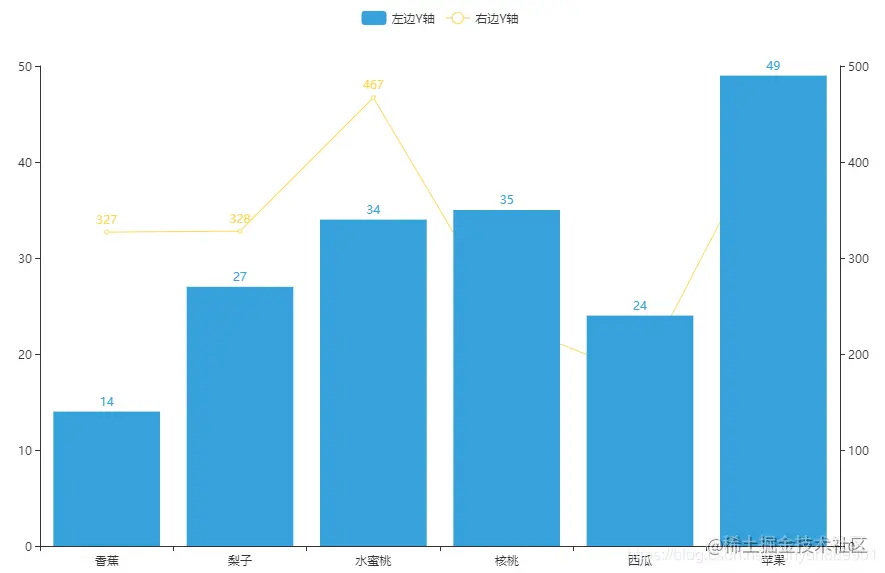``````from pyecharts.charts import *
from pyecharts import options as opts
import random

x_data = [' Banana ', ' pears ', ' honey peach ', ' walnut ', ' watermelon ', ' Apple ']
y_data_1 = [random.randint(10, 50) for _ in range(len(x_data))]
y_data_2 = [random.randint(100, 500) for _ in range(len(x_data))]

def bar_line_combine_with_two_axis():
bar = Bar(init_opts=opts.InitOpts(theme='light',
width='1000px',
height='600px'))
bar.extend_axis(yaxis=opts.AxisOpts())
bar.add_yaxis(' On the left Y Axis ', y_data_1, yaxis_index=0)

line = Line(init_opts=opts.InitOpts(theme='light',
width='1000px',
height='600px'))
#  take line Data is passed through yaxis_index Point to the... Added after Y Axis
line.add_yaxis(' On the right Y Axis ', y_data_2, yaxis_index=1)

bar.overlap(line)
return bar

chart = bar_line_combine_with_two_axis()
chart.render_notebook()
Copy code ``````

## 5. Histogram —— double Y Axis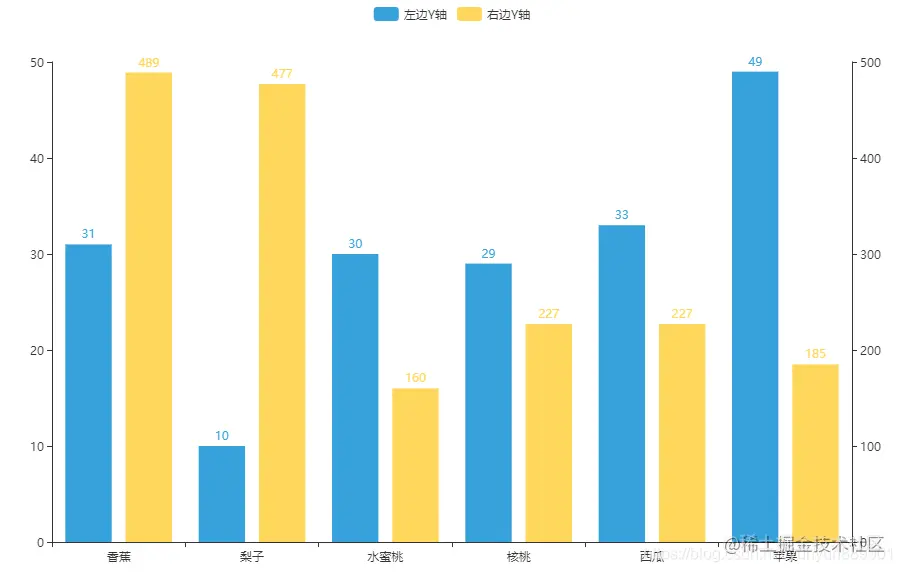``````from pyecharts.charts import *
from pyecharts import options as opts
import random

x_data = [' Banana ', ' pears ', ' honey peach ', ' walnut ', ' watermelon ', ' Apple ']
y_data_1 = [random.randint(10, 50) for _ in range(len(x_data))]
y_data_2 = [random.randint(100, 500) for _ in range(len(x_data))]

def bar_with_multiple_axis():
bar = Bar(init_opts=opts.InitOpts(theme='light',
width='1000px',
height='600px'))
bar.extend_axis(yaxis=opts.AxisOpts())
#  Specify the... Used separately Y Axis
bar.add_yaxis(' On the left Y Axis ', y_data_1, yaxis_index=0)
bar.add_yaxis(' On the right Y Axis ', y_data_2, yaxis_index=1)
return bar

chart = bar_with_multiple_axis()
chart.render_notebook()
Copy code ``````

## 6. Broken line diagram —— double X Axis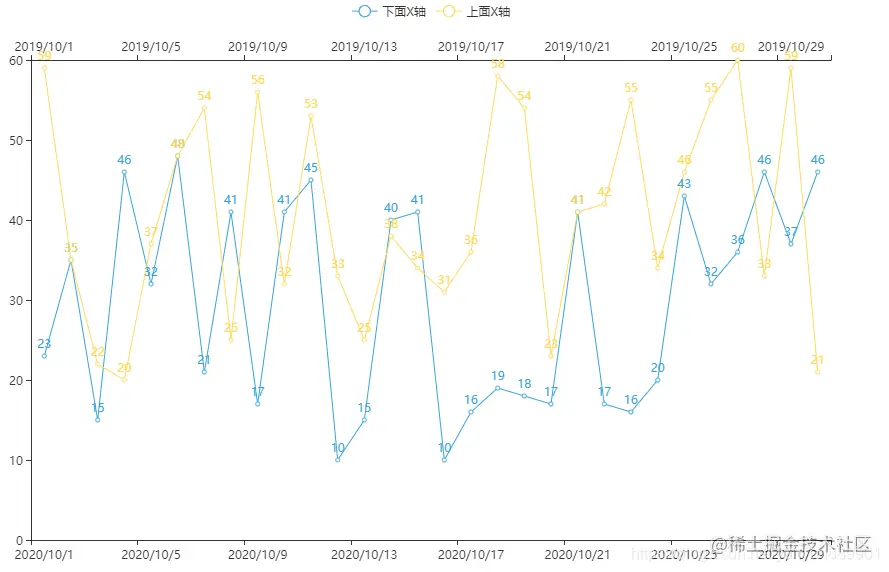``````from pyecharts.charts import *
from pyecharts import options as opts
import random

x_data_1 = ["2020/10/{}".format(i + 1) for i in range(30)]
x_data_2 = ["2019/10/{}".format(i + 1) for i in range(30)]
y_data_1 = [random.randint(10, 50) for _ in range(30)]
y_data_2 = [random.randint(20, 60) for _ in range(30)]

def line_with_two_xaxis():
line = Line(init_opts=opts.InitOpts(theme='light',
width='1000px',
height='600px'))
line.extend_axis(xaxis_data=x_data_2, xaxis=opts.AxisOpts())
line.add_yaxis(' below X Axis ', y_data_1)
line.add_yaxis(' above X Axis ', y_data_2)
return line

chart = line_with_two_xaxis()
chart.render_notebook()
Copy code ``````

## 7. Abbreviated axis ——inside Components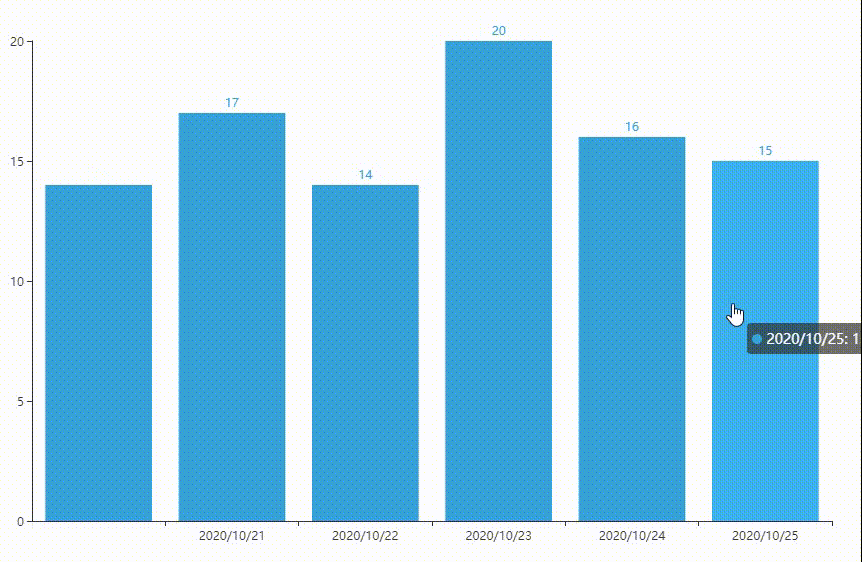``````from pyecharts.charts import *
from pyecharts import options as opts
import random

x_data = ["2020/10/{}".format(i + 1) for i in range(30)]

#  Randomly generate point data
y_data = [random.randint(10, 20) for i in range(len(x_data))]

def bar_datazoom_inside():
bar = Bar(init_opts=opts.InitOpts(theme='light',
width='1000px',
height='600px'))
bar.set_global_opts(datazoom_opts=opts.DataZoomOpts(type_='inside',
range_start=50,   #  Set the start stop position ,50%-100%
range_end=100))
return bar

chart = bar_datazoom_inside()
chart.render_notebook()
Copy code ``````

## 8. Abbreviated axis ——slider Components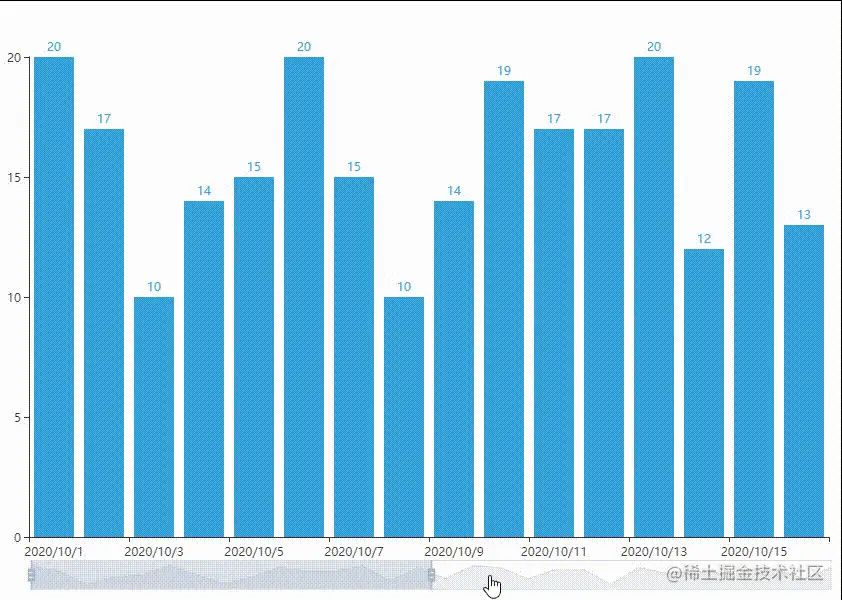``````from pyecharts.charts import *
from pyecharts import options as opts
import random

x_data = ["2020/10/{}".format(i + 1) for i in range(30)]

#  Randomly generate point data
y_data = [random.randint(10, 20) for i in range(len(x_data))]

def bar_with_datazoom_slider():
bar = Bar(init_opts=opts.InitOpts(theme='light',
width='1000px',
height='600px'))
bar.set_global_opts(datazoom_opts=opts.DataZoomOpts(range_start=50,   #  Set the start stop position ,50%-100%
range_end=100))
return bar

chart = bar_with_datazoom_slider()
chart.render_notebook()
Copy code ``````

## 9.XY The axis turns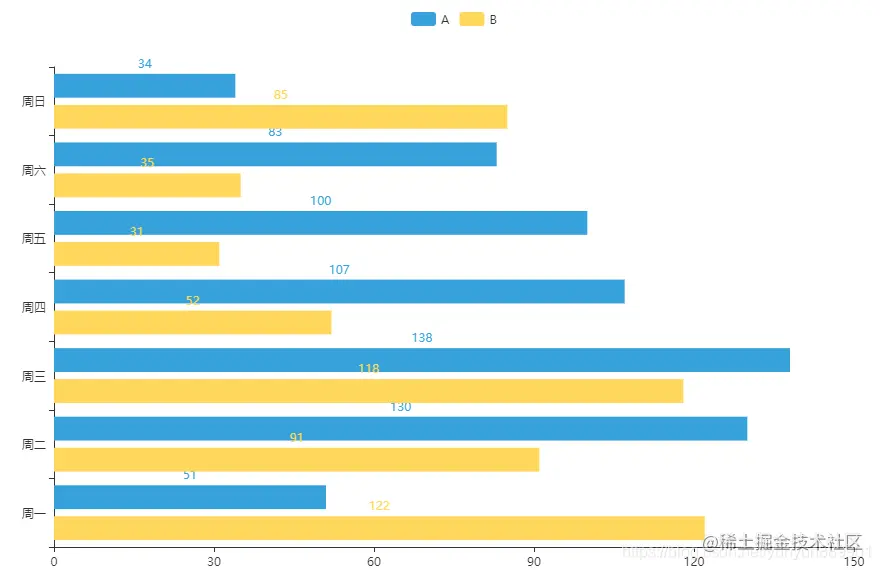``````from pyecharts.charts import *
from pyecharts import options as opts
from pyecharts.faker import Faker

def bar_reverse_axis():
bar = Bar(init_opts=opts.InitOpts(theme='light',
width='1000px',
height='600px'))
# x,y The axis turns
bar.reversal_axis()
return bar

chart = bar_reverse_axis()
chart.render_notebook()
Copy code ``````

## 10. Animate

There will be an animation effect before the chart is loaded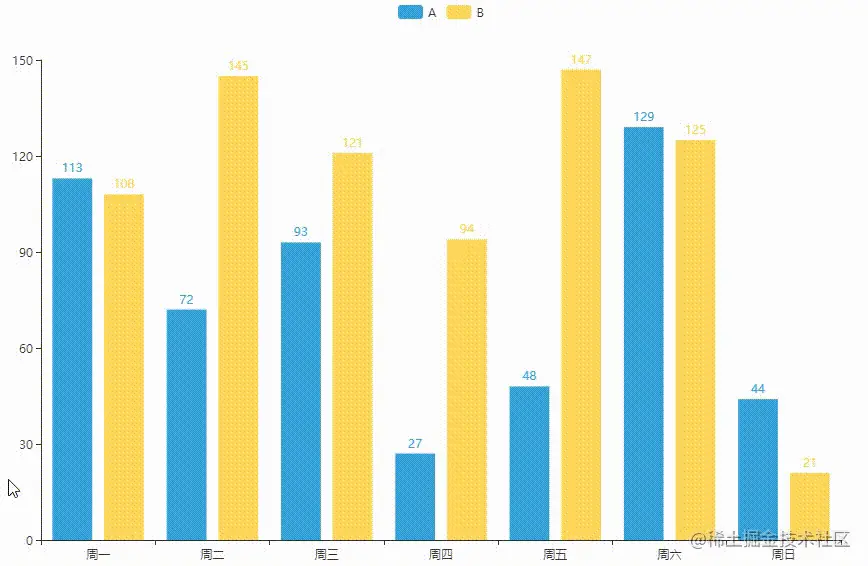``````from pyecharts.charts import *
from pyecharts import options as opts
from pyecharts.faker import Faker

"""  For more animation effects, see ：https://echarts.apache.org/examples/zh/editor.html?c=line-easing """

def bar_with_animation():
bar = Bar(init_opts=opts.InitOpts(theme='light',
width='1000px',
height='600px',
animation_opts=opts.AnimationOpts(animation_delay=1000,   #  Animation Offset
animation_easing='bounceIn')
)
)
return bar

chart = bar_with_animation()
chart.render_notebook()
Copy code ``````## 11. Histogram with visual components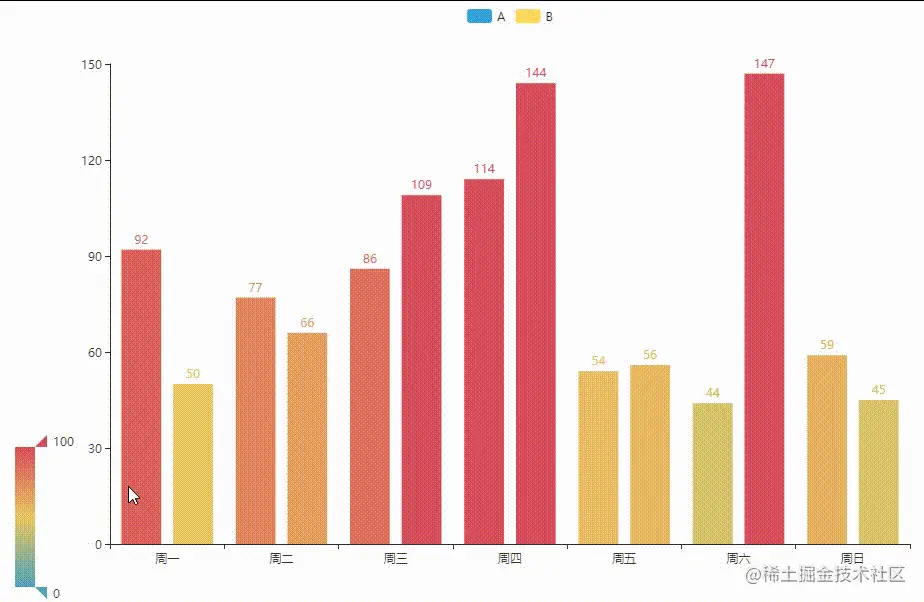``````from pyecharts.charts import *
from pyecharts import options as opts
from pyecharts.faker import Faker

def bar_with_visualmap_color():
bar = Bar(init_opts=opts.InitOpts(theme='light',
width='1000px',
height='600px'))
#  Set the visual components , Data is mapped by color by default , The range of values is 0-100, It can be done by min_ and max_ Customize
bar.set_global_opts(visualmap_opts=opts.VisualMapOpts())
return bar

chart = bar_with_visualmap_color()
chart.render_notebook()
Copy code ``````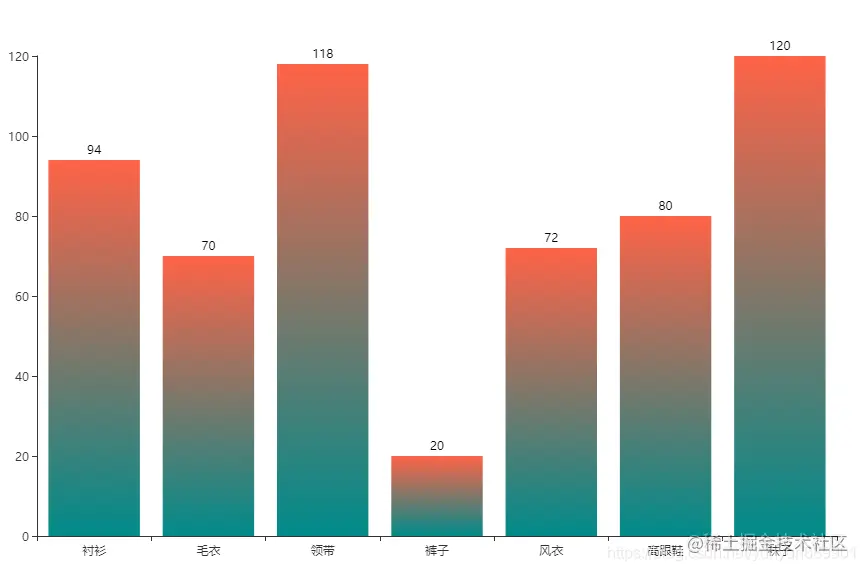``````from pyecharts.charts import *
from pyecharts import options as opts
from pyecharts.faker import Faker
from pyecharts.commons.utils import JsCode

color_js = """ new echarts.graphic.LinearGradient( 0, 1, 0, 0, [{offset: 0, color: '#008B8B'}, {offset: 1, color: '#FF6347'}], false) """

bar = Bar(init_opts=opts.InitOpts(theme='light',
width='1000px',
height='600px'))
#  Use JsCode Execute gradient code
itemstyle_opts=opts.ItemStyleOpts(color=JsCode(color_js)))

return bar

chart.render_notebook()
Copy code ``````

If it helps you in your study, you must keep it , More information about Python Learning experience , Tool installation package sharing , But I don't know , Pay attention to me , Continuous updating .